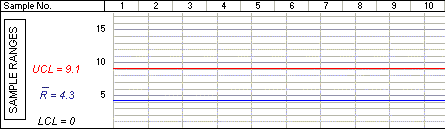# Chart Plotting Exercise (5)

The next step is to find the range of the items in the sample. This is found by subtracting the weight of the lightest item from that of the heaviest:

Range = 178 - 175 = 3

You should then add this value to the last box in the first column as shown below.

Date 01/04 01:00 177 176 178 177 175 883 177 3

You then add this value to your chart. This range value of 3 is then plotted on the R graph at the point where the first vertical line meets the horizontal line representing the value of 3. Do this now on the graph below, by clicking the correct point.